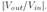### Create an Account

Home / Questions / A circuit contains two coincident equal poles at ωp1 Construct the Bode plot of An ampli...

# A circuit contains two coincident equal poles at ωp1 Construct the Bode plot of An amplifier exhibits two poles at 100 MHz and 10 GHz and a zero at 1 GHz Construct the Bode plot of

A circuit contains two coincident (i.e., equal) poles at ωp1. Construct the Bode plot of2. An amplifier exhibits two poles at 100 MHz and 10 GHz and a zero at 1 GHz. Construct the Bode plot of3. An ideal integrator contains a pole at the origin, i.e., ωp = 0. Construct the Bode plot ofWhat is the gain of the circuit at arbitrarily low frequencies?

Apr 24 2020 View more View LessSubscribe To Get Solution